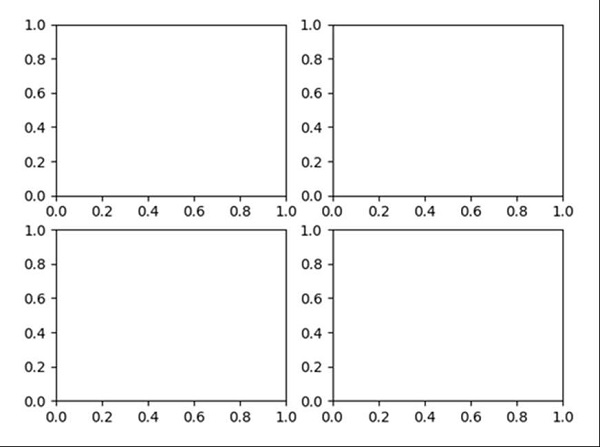# How to make several plots on a single page using Matplotlib(Python)?

Using plt.figure(), we can create a figure and can split that in parts using subplot(221) methods, where nrows=2, nlos=2, and (1,2,3,4) represent the index position.

## Steps

• Create a new figure, or activate an existing figure, using figure() method.

• Add a subplot to the current figure, using subplot() method, where nrows = 2, ncols = 2 and index = 1.

• Add a subplot to the current figure, using subplot() method, where nrows = 2, ncols = 2 and index = 2.

• Add a subplot to the current figure, using subplot() method, where nrows = 2, ncols = 2 and index = 3.

• Add a subplot to the current figure, using subplot() method, where nrows = 2, ncols = 2 and index = 4

• To show the figure, we can use plt.show() method.

## Example

from matplotlib import pyplot as plt

fig = plt.figure()
plt.subplot(221)
plt.subplot(222)
plt.subplot(223)
plt.subplot(224)
plt.show()

## Output